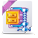DX Application Performance Management

View Only

Historical Data Calculation of Calculator outputsGeethu JohnDec 22, 2015 02:46 AMHallett GermanDec 22, 2015 09:44 AMGeethu JohnDec 22, 2015 10:31 PMMatthew MuskaloonDec 22, 2015 10:20 AMThomas WyszomierskiDec 22, 2015 02:07 PMGeethu JohnDec 22, 2015 10:46 PMHallett GermanDec 23, 2015 08:54 AM• 1.  Historical Data Calculation of Calculator outputs

Posted Dec 22, 2015 02:46 AM

Hi all ,

Has anyone faced discrepancies in values/outputs of calculators, when historical view is taken and  resolution is increased?

Suppose I have a metric in Investigator :

Say MethodA - The ART values for 8 data points are 0,0,0,3044,0,0,3132,2942.

I created a simple sum calculator out of this which will in turn give me the same value.

I created a java-script calculator to average the response time of MethodA and MethodB which will have a different data set. Suppose MethodB ART is always zero , then java script calculator should be giving me the same output as MethodA ART.

So I have three graphs now. MethodA , Simple Calculator and Javascript Calculator.

If I click on historical view and set the resolution as 1 minute , the above 8 data points will be averaged to 2 points. But the values of those 2 data points in the all the three graphs are different.

(According to me, it should be giving me the same output).

Method A gives - 3044 and 3037 -------> (3044/1 and (3132+2942)/2). Here , only those data points > 0 are considered for average calculation , which makes sense.

Simple Calculator - 3044 and 3132 ( Here it considers the data point which has the maximum value )

Java script calculator - 761 and 1519 ( Here it just takes all the points and divide by 4. (0+0+0+3444)/4 and (0+0+3132+2942)/4.

Am I missing anything ? Or this is how calculators behave when historical values are taken?

Thanks

Geethu

• 2.  Re: Historical Data Calculation of Calculator outputs

Posted Dec 22, 2015 09:44 AM

Hi Geethu:

What version are running and can you attach the Javascript calculator?

Thanks

Hal German

• 3.  Re: Historical Data Calculation of Calculator outputs

Posted Dec 22, 2015 10:31 PM

Hi Hal,

I am running 9.5.2..

Attaching the script :

Attachment(s)OperationART.txt.zip   1 KB 1 version

• 4.  Re: Historical Data Calculation of Calculator outputs

Posted Dec 22, 2015 10:20 AM

Hi Geethu,

1. What type of calculator are you using?  Sum, Average, Min or Max?

2. What is your time frame that you are comparing.  You have the resolution at 1 minute, but what is your time range?

Smartstor tiering can affect this if your time range goes beyond the first tier.

Matt

• 5.  Re: Historical Data Calculation of Calculator outputs

Posted Dec 22, 2015 02:07 PM

It would also be interesting to know if you are using a Counter or Interval Counter for your calculator. Interval Counters will sum values aggregated over time while a counter will return the highest value over the interval. For older metrics, smartstor tiers can also create inconsistencies between metrics and calculators derived by those metrics.

• 6.  Re: Historical Data Calculation of Calculator outputs

Posted Dec 22, 2015 10:46 PM

Hi Thomas,

I use counter for my calculators as the input was response times. I tried interval counter,but as you said , it just sums up everything in that interval.

Just wondering , how the metrics in Investigator tree show up everything so beautifully when we take different averages.

Is it a different logic or algorithm that gets applied when we create calculators?

Geethu

• 7.  Re: Historical Data Calculation of Calculator outputs

Posted Dec 23, 2015 08:54 AM

Geethu:

In talking with Matt, the best course of action would be open a case to resolve.

Thanks

Hal German

• 8.  Re: Historical Data Calculation of Calculator outputs

Posted Dec 22, 2015 10:38 PM

Hi Matt,

The simple Introscope calculator that I use is sum operation. Because I need the sum of response times of few processes

I take the sum of say 5 processes from server 1 and similarly take the sum of similar 5 processes from server 2. So I use two simple calculators here.Then I use a JS calculator to take the average so that it will not count datapoints with 0 value for average calculation.

Also , the time frame is 8 minutes only.

Geethu